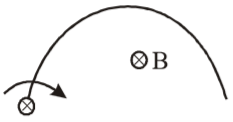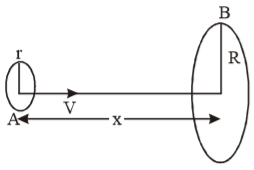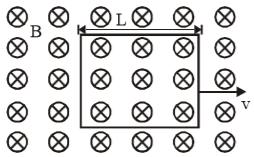A semicircular wire of radius R is rotated with constant angular velocity $\mathrm{\omega }$ about an axis passing through one end and perpendicular to the plane of the wire.There is a uniform magnetic field of strength B. The induced emf between the ends is -

1. ${\mathrm{B\omega R}}^{2}/2$

2. $2{\mathrm{B\omega R}}^{2}$

3. is variable

4. none of these

Concept Questions :-

Motional emf
High Yielding Test Series + Question Bank - NEET 2020

Difficulty Level:

The inductance of a closed-packed coil of 400 turns is 8 mH. A current of 5 mA is passed through it. The magnetic flux through the coil is approximately

1.

2.

3.

4.

Concept Questions :-

Self-inductance
High Yielding Test Series + Question Bank - NEET 2020

Difficulty Level:

Loop A of radius (r << R) moves towards loop B with a constant velocity v in such a way that their planes are always parallel.What is the distance (x) between the two loops when the induced emf in loop A is maximum –

1. $\mathrm{R}$

2. $\frac{\mathrm{R}}{\sqrt{2}}$

3. $\frac{\mathrm{R}}{2}$

4. $\mathrm{R}\left(1-\frac{1}{\sqrt{2}}\right)$

Concept Questions :-

Motional emf
High Yielding Test Series + Question Bank - NEET 2020

Difficulty Level:

The current in an L – R circuit builds up to ${\left(3/4\right)}^{\mathrm{th}}$ of its steady state value in 4 seconds. The time constant of this circuit is

1.

2.

3.

4.

Concept Questions :-

LR circuit
High Yielding Test Series + Question Bank - NEET 2020

Difficulty Level:

The magnetic flux through each turn of a 100 turn coil is , where t is in second. The induced emf at t = 2 s is

1.

2.

3.

4.

Concept Questions :-

Faraday 's law and lenz law
High Yielding Test Series + Question Bank - NEET 2020

Difficulty Level:

An emf of 15 volt is applied in a circuit containing 5 henry inductance and 10 ohm resistance. The ratio of the currents at time t = $\infty$ and at t = 1 second is -

1.  $\frac{{\mathrm{e}}^{1/2}}{{\mathrm{e}}^{1/2}-1}$

2.  $\frac{{\mathrm{e}}^{2}}{{\mathrm{e}}^{2}-1}$

3.  $1-{\mathrm{e}}^{-1}$

4.  ${\mathrm{e}}^{-1}$

Concept Questions :-

LR circuit
High Yielding Test Series + Question Bank - NEET 2020

Difficulty Level:

A circular coil of radius 5 cm has 500 turns of a wire. The approximate value of the coefficient of self induction of the coil will be -

1.

2.

3.

4.

Concept Questions :-

Self-inductance
High Yielding Test Series + Question Bank - NEET 2020

Difficulty Level:

A coil of radius 1 cm and  turns 100 is placed in the middle of a long solenoid of radius 5 cm and having 8 turns/cm. The mutual induction in millihenry will be-

1.  0.0316

2.  0.063

3.  0.105

4.  Zero

Concept Questions :-

Mutual-inductance
High Yielding Test Series + Question Bank - NEET 2020

Difficulty Level:

A conducting square loop of side L and resistance R moves in its plane with a uniform velocity v perpendicular to one of its sides.A magnetic induction B, constant in time and space, pointing perpendicular and into the plane of the loop exists everywhere, see figure. The current induced in the loop is

1.  BLv/R clockwise

2.  BLv/R anticlockwise

3.  2 BLv/R anticlockwise

4.  zero

Concept Questions :-

Motional emf
High Yielding Test Series + Question Bank - NEET 2020

Difficulty Level:

A copper rod of length 0.19 m is moving parallel to a long wire with a uniform velocity of 10 m/s. The long wire carries 5 ampere current and is perpendicular to the rod. The ends of the rod are at distances 0.01 m and 0.2 m from the wire. The emf induced in the rod will be-

1.

2.

3.

4.

Concept Questions :-

Motional emf